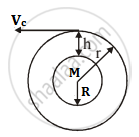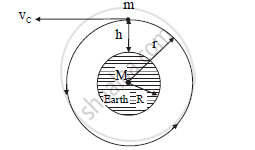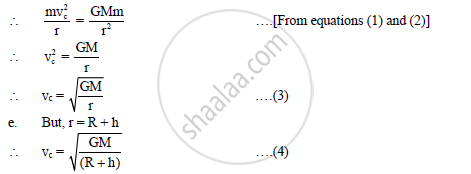Derive an Expression for Critical Velocity of a Satellite Revolving Around the Earth in a Circular Orbit. - Physics

Derive an expression for critical velocity of a satellite revolving around the earth in a circular orbit.

Obtain an expression for critical velocity of a satellite orbiting around the earth

Solution 1Consider a satellite of mass m revolving round the Earth at a height ‘h’ above the surface of the Earth.
Let M be the mass and R be the radius of the Earth.
The satellite is moving with velocity Vc and the radius of the circular orbit is r = R + h.

Centripetal force = Gravitational force

:.(mV_c^2)/r=(GMm)/r^2

:.V_c^2=GM/r

:.V_c=sqrt((GM)/(R+h)) ...............(Equ. 1)

This is the expression for critical velocity of a satellite moving in a circular orbit around the Earth.

We know that,

g_h=(GM)/((R+h)^2

:.GM=g_h(R+h)^2

Substituting in equation 1, we get

:.V_c=sqrt(g_h(R+h)^2/(R+h)

:.V_c=sqrt(g_h(R+h)

where gh is the acceleration due to gravity at a height h above the surface of the Earth.

Solution 2Let,
M = mass of the earth
R = radius of the earth
h = height of the satellite from the earth’s surface
m = mass of the satellite
vc
= critical velocity of the satellite in the given orbit
r = (R + h) = radius of the circular orbit

For the circular motion of the satellite, the necessary centripetal force is given as

F_CP = (mv_c^2)/r           1

Gravitational force provides the centripetal force necessary for the circular motion of the satellite

∴ FCP = FGEquation (4) represents the expression for critical velocity.

Concept: Acceleration Due to Gravity and Its Variation with Altitude and Depth
Is there an error in this question or solution?